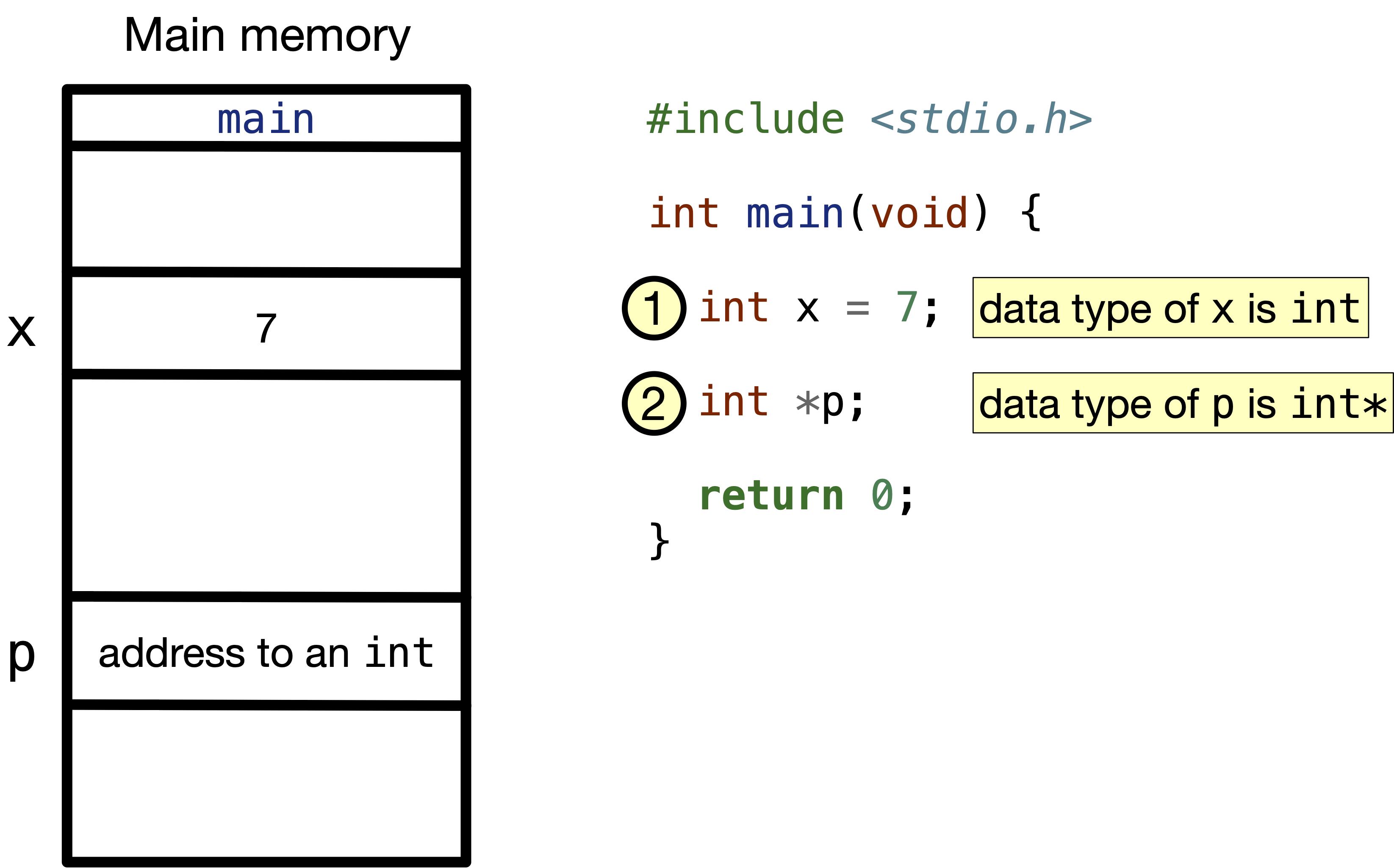# 6.2. What are pointers?#

As we discussed earlier, every variable in our code is stored in the main memory. A pointer is a variable that holds the address of another variable. In the following figure, we have two variables: a int variable named x and is set to $$7$$, and a pointer to a int named p that holds an address to a int.Fig. 6.2 int and pointer to int (int*) variables in code and memory.#

As we learned before, declaring a variable without initializing it makes the variable hold a garbage value, i.e. in the above program, the variable p holds a garbage address.

How do we assign an address to a pointer? Using the reference operator &, we can get the address of a variable. For example, &x gets the address of variable x, which is $$2$$ in the following figure. Then, we can assign this address $$2$$ to p as in the following code.

How do we get the value stored in an address? Using the dereference operator * on a pointer variable, we can get the value stored at an address. For example, *p gets the value stored at address $$2$$, which is equal to $$7$$.

Deep-dive Let’s look a little closer on the addresses and values stored in some addresses. In the following code, we print addresses and values stored in ints x and y, and in int* p. A format specifier for a pointer is %p. Download reference-deference.c if you want to play with the code yourself.

Code

#include <stdio.h>

int main(void) {
int x = 7;
int *p;
p = &x;
int y;
y = *p;

printf("Address of x: %p\n Value of x: %d\n", &x, x);
printf("Address of p: %p\n Value of p: %p\n", &p, p);
printf("Address of y: %p\n Value of y: %d\n", &y, y);
printf("Value stored in address %p is %d\n", p, *p);

return 0;
}


Potential Output

Address of x: 0x30e2af178
Value of x: 7
Value of p: 0x30e2af178
Value of y: 7
Value stored in address 0x30e2af178 is 7


Key observations from the printed statements:

1. The variable x and y are stored in different places, but have the same value $$7$$.

2. The pointer variable p has a different address from the address it stores. This is pointer p has the nature of storing addresses.

3. * gets us the value stored at an address, and & gets us the address of a variable, even if the variable is a pointer. This would mean *(&x) would mean x, as * and & cancel each other.

## 6.2.1. Tracing exercise#

Let’s trace the code below to get a better understanding of how pointers work. A short video that goes over a program with pointers line by line is posted below. We dive deeper into what happens in the main memory as we execute the program.

Code

#include <stdio.h>

int main(void) {
int x = 3, y = 4;
int *a, *b;

a = &x;
b = &y;
printf("Before operations:\n");
printf("Value of x: %d\n", x);
printf("Value of y: %d\n", y);
printf("Value at address a: %d\n", *a);
printf("Value at address b: %d\n", *b);

*a = 6;
*b = *b + 3;
printf("After operations:\n");
printf("Value of x: %d\n", x);
printf("Value of y: %d\n", y);
printf("Value at address a: %d\n", *a);
printf("Value at address b: %d\n", *b);
return 0;
}


Output

Before operations:
Value of x: 3
Value of y: 4